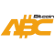Bitcoin ABC  0.22.12 P2P Digital Currency
hash.h File Reference
`#include <crypto/common.h>`
`#include <crypto/ripemd160.h>`
`#include <crypto/sha256.h>`
`#include <prevector.h>`
`#include <serialize.h>`
`#include <uint256.h>`
`#include <version.h>`
`#include <vector>`
Include dependency graph for hash.h:
This graph shows which files directly or indirectly include this file:

Go to the source code of this file.

## Classes

class  CHash256
A hasher class for Bitcoin's 256-bit hash (double SHA-256). More...

class  CHash160
A hasher class for Bitcoin's 160-bit hash (SHA-256 + RIPEMD-160). More...

class  CHashWriter
A writer stream (for serialization) that computes a 256-bit hash. More...

class  CHashVerifier< Source >
Reads data from an underlying stream, while hashing the read data. More...

## Typedefs

typedef uint256 ChainCode

## Functions

template<typename T1 >
uint256 Hash (const T1 pbegin, const T1 pend)
Compute the 256-bit hash of an object. More...

template<typename T1 , typename T2 >
uint256 Hash (const T1 p1begin, const T1 p1end, const T2 p2begin, const T2 p2end)
Compute the 256-bit hash of the concatenation of two objects. More...

template<typename T1 >
uint160 Hash160 (const T1 pbegin, const T1 pend)
Compute the 160-bit hash an object. More...

uint160 Hash160 (const std::vector< uint8_t > &vch)
Compute the 160-bit hash of a vector. More...

template<unsigned int N>
uint160 Hash160 (const prevector< N, uint8_t > &vch)
Compute the 160-bit hash of a vector. More...

template<typename T >
uint256 SerializeHash (const T &obj, int nType=SER_GETHASH, int nVersion=PROTOCOL_VERSION)
Compute the 256-bit hash of an object's serialization. More...

uint32_t MurmurHash3 (uint32_t nHashSeed, const std::vector< uint8_t > &vDataToHash)

void BIP32Hash (const ChainCode &chainCode, uint32_t nChild, uint8_t header, const uint8_t data, uint8_t output)

## ◆ ChainCode

 typedef uint256 ChainCode

Definition at line 19 of file hash.h.

## ◆ BIP32Hash()

 void BIP32Hash ( const ChainCode & chainCode, uint32_t nChild, uint8_t header, const uint8_t data, uint8_t output )

Definition at line 72 of file hash.cpp.

Here is the call graph for this function:
Here is the caller graph for this function:

## ◆ Hash() [1/2]

template<typename T1 >
 uint256 Hash ( const T1 pbegin, const T1 pend )
inline

Compute the 256-bit hash of an object.

Definition at line 72 of file hash.h.

Here is the call graph for this function:
Here is the caller graph for this function:

## ◆ Hash() [2/2]

template<typename T1 , typename T2 >
 uint256 Hash ( const T1 p1begin, const T1 p1end, const T2 p2begin, const T2 p2end )
inline

Compute the 256-bit hash of the concatenation of two objects.

Definition at line 84 of file hash.h.

Here is the call graph for this function:

## ◆ Hash160() [1/3]

template<typename T1 >
 uint160 Hash160 ( const T1 pbegin, const T1 pend )
inline

Compute the 160-bit hash an object.

Definition at line 98 of file hash.h.

Here is the call graph for this function:
Here is the caller graph for this function:

## ◆ Hash160() [2/3]

 uint160 Hash160 ( const std::vector< uint8_t > & vch )
inline

Compute the 160-bit hash of a vector.

Definition at line 109 of file hash.h.

Here is the call graph for this function:

## ◆ Hash160() [3/3]

template<unsigned int N>
 uint160 Hash160 ( const prevector< N, uint8_t > & vch )
inline

Compute the 160-bit hash of a vector.

Definition at line 115 of file hash.h.

Here is the call graph for this function:

## ◆ MurmurHash3()

 uint32_t MurmurHash3 ( uint32_t nHashSeed, const std::vector< uint8_t > & vDataToHash )

Definition at line 13 of file hash.cpp.

Here is the call graph for this function:
Here is the caller graph for this function:

## ◆ SerializeHash()

template<typename T >
 uint256 SerializeHash ( const T & obj, int nType = `SER_GETHASH`, int nVersion = `PROTOCOL_VERSION` )

Compute the 256-bit hash of an object's serialization.

Definition at line 196 of file hash.h.

Here is the call graph for this function:
Here is the caller graph for this function: